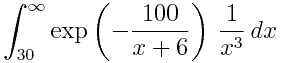# Numerical integration trick

Suppose you want to evaluate the following integral:We’d like to do a change of variables to make the range of integration finite, and we’d like the transformed integral to be easy to evaluate numerically.

The change of variables t = 1/x2 transforms the range of integration from (30, ∞) to (0, 1/900). I’ll explain where this choice came from and why it results in a nice function to integrate.

The integrand is essentially 1/x3 for large x because the exponential term approaches 1. If the integrand were exactly 1/x3 then the change of variables t = 1/x2 would make the integrand constant. So we’d expect this change of variables to give us an integrand that behaves well. In particular, the integral should be bounded at 0.

(If you’re not careful, a change of variables may just swap one kind of improper integral for another. That is, you can make the domain of integration finite, but at the cost of introducing a singularity in the transformed integrand. However, we don’t have that problem here because our change of variables was just right.)

In general, if you need to integrate a function that behaves like 1/xn as x goes to infinity, try the change of variables t = 1/xn-1.

Related post: The care and treatment of singularities

## 6 thoughts on “Numerical integration trick”

1. Can you be more specific about what 1 = 1/x^2 means? I know that I’m probably being stupid, but to me that looks like x = +/-1, which is outside the stated bounds of your integral.

2. Jitse Niesen

I’m pretty sure that 1 = 1/x^2 is a typo for y = 1/x^2, where y is your new variable that you integrate over.

3. Sorry about the typo. I meant the new variable t = 1/x^2.

4. Good one. I learnt integration at school, so I forgot most of it. Cool

5. Jan Van lent

Other transformations that work are t = -100/(x+6) or t=30/x.

6. Ok, that’s what I thought. But you did it three times so I figured I was missing some kind of notation that I was unfamiliar with. :-D

@Jan: true, but they don’t leave it as flat as John’s, which I think is the point of using 1/x^2.